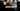# Leetcode | Solution of Intersection of Two Arrays in JavaScript

May 10th, 2020
|

In this post, we will solve the Intersection of Two Arrays from leetcode and compute it's time and space complexities. Let's begin.

# Problem Statement

The question can be found at leetcode Intersection of Two Arrays problem.

The question states that we are given two arrays and we need to find their intersection i.e. find numbers that are in both arrays.

# Constraints and challenges

The question gives the following constraints

• All numbers in the result must be unique
• The numbers in the result can be in any order

# Solution

The given constraints in the question make our job easier. Since we are given two arrays that may have duplicates, we can save both of them in two sets. Once we do that, set will get rid of duplicates automatically and we are left with unique values.

Next, we can loop over one set and find how many of the numbers in set 1 is also present in set 2. We keep on pushing these values in an array and once we are done with the loop, we have our answer array.

We have discussed the approach, I urge you to go ahead on leetcode and give it another try.If you are here, it means something went wrong in implementation or you are just too lazy. In any case, let's see a simple implementation of the above logic.
``````var intersection = function (nums1, nums2) {
const set1 = new Set(nums1);
const set2 = new Set(nums2);

const arr = [];

for (let val of set1) {
if (set2.has(val)) {
arr.push(val)
}
}

return arr;
};``````

Nothing very fancy in the code. Very straight forward implementation of the logic discussed above.

Here are the stats on submission

``````Status: Accepted
Runtime: 56ms
Memory: 34.8MB``````

## Time and space complexity

### Time complexity

We are looping over the set once, so time complexity would be O(n).

### Space complexity

We are using extra space for sets and array, but all of them would grow linearly with respect to inputs. So space complexity is also linear, O(n).

# Summary

So, we solved the Intersection of Two Arrays problem by using two sets and a loop. Finally, we calculated the time and space complexities.

I hope you enjoyed solving this question. This is it for this one, complete source code for this post can be found on my Github Repo. Will see you in the next one.